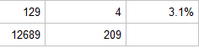## proc report

Hi!

I am using PROC REPORT to create a report on a table I created using PROC SQL. Everything is working fine, however I am trying to determine how to populate the last cell. Here is my current code:

``````proc sql;
create table tbl_AP as
select
Grll,
count(PI) as Total,
count (AR) as Inis,
calculated Inis / Calculated Total as Return_Rate
from db.	where GROUP= 3
group by Grll;
quit;

proc report data= tbl_AP
Title1 "Distribution of People";
column ('Groups' Grll) ('Employees' Total Inis) ('Return Rate' Return_Rate);
define Gll / display 'Gr-Level';
define Total / sum;
define inis / sum;
define Return_Rate/ display '%' format=percent8.1;
rbreak after /dol skip summarize;
compute after;
Grll = 'Total';
endcomp;
run;``````

The entire table prints correctly, however the last row (the totals row) leaves a blank where the return rate column is calculated. Here is a partial snippet, however I am unable to insert the entire table output due to privacy policies.Thank you!

2 REPLIES 2

## Re: proc report

Hello,

I don't know if this is the best area for your post (this is for graphics), but I think I have a simple solution for your issue.  I don't know how well RBREAK works outside of the listing destination, but I don't think you even need to use PROC REPORT in this case:

``````proc sql;
create table tbl_AP as
select
Grll,
count(PI) as Total,
count (AR) as Inis,
calculated Inis / Calculated Total as Return_Rate
from db.	where GROUP= 3
group by Grll

OUTER UNION CORR
select 'Total' as grll,sum(total) as total,sum(inis) as inis,sum(return_rate) as return_rate          from (select Grll,
count(PI) as Total,
count (AR) as Inis,
calculated Inis / Calculated Total as Return_Rate
from db.	where GROUP= 3        group by grll) ;
quit;``````

OUTER UNION CORR matches columns by their variable names.  I take your original query and add it as an inline view for the second query and then aggregate across that using SQL.  I don't know how you wanted return_rate to be aggregated (sum, mean, etc.) so you can change that as needed.

If all you wanted for the total row was to do the same query without grouping you would do the following:

``````proc sql;
create table tbl_AP as
select
Grll,
count(PI) as Total,
count (AR) as Inis,
calculated Inis / Calculated Total as Return_Rate
from db.	where GROUP= 3
group by Grll

OUTER UNION CORR
select 'Total' as Grll,
count(PI) as Total,
count (AR) as Inis,
calculated Inis / Calculated Total as Return_Rate
from db.	where GROUP= 3
;
quit;``````

## Re: proc report

DISPLAY variables aren't summarized. And the fun part is none of the basic summary functions work properly with percentages (or other rates) unless the denominators are exactly the same for each group.

Discussion stats
• 2 replies
• 534 views
• 0 likes
• 3 in conversation